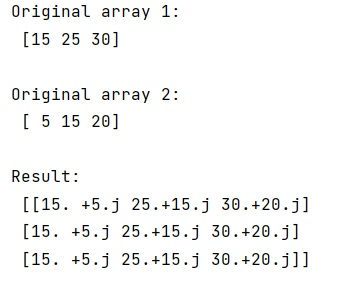# NumPy: Creating a complex array from 2 real ones?

Learn, how to create a complex array from 2 real ones in Python NumPy?
Submitted by Pranit Sharma, on January 17, 2023

NumPy is an abbreviated form of Numerical Python. It is used for different types of scientific operations in python. Numpy is a vast library in python which is used for almost every kind of scientific or mathematical operation. It is itself an array which is a collection of various methods and functions for processing the arrays.

## Creating a complex array from 2 real ones

Suppose that we are given two real arrays (a and b), and we need to create a complex array (c) that takes the two real arrays as their real and imaginary parts respectively.

For this purpose, we will first create an empty NumPy array of some specific shape and also, and we will define the data type of this array as complex128.

Then we will assign the real and imaginary parts of this array as A and B.

Let us understand with the help of an example,

## Python code to create a complex array from 2 real ones

```# Import numpy
import numpy as np

# Import pandas
import pandas as pd

# Creating two numpy arrays
arr1 = np.array([15, 25, 30])
arr2 = np.array([5, 15, 20])

# Display original arrays
print("Original array 1:\n",arr1,"\n")
print("Original array 2:\n",arr2,"\n")

# Creating a complex array
com = np.empty((3,3),dtype=np.complex128)
com.real = arr1
com.imag = arr2

# Display result
print("Result:\n",com)
```

Output:What's New (MCQs)

Top Interview Coding Problems/Challenges!

IncludeHelp's Blogs

Languages: » C » C++ » C++ STL » Java » Data Structure » C#.Net » Android » Kotlin » SQL
Web Technologies: » PHP » Python » JavaScript » CSS » Ajax » Node.js » Web programming/HTML
Solved programs: » C » C++ » DS » Java » C#
Aptitude que. & ans.: » C » C++ » Java » DBMS
Interview que. & ans.: » C » Embedded C » Java » SEO » HR
CS Subjects: » CS Basics » O.S. » Networks » DBMS » Embedded Systems » Cloud Computing
» Machine learning » CS Organizations » Linux » DOS
More: » Articles » Puzzles » News/Updates